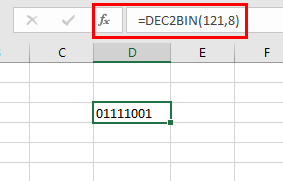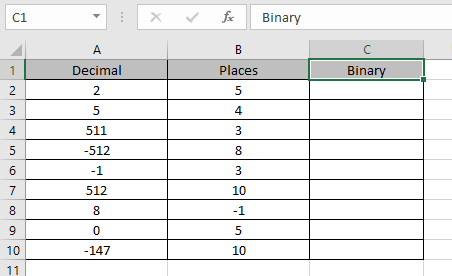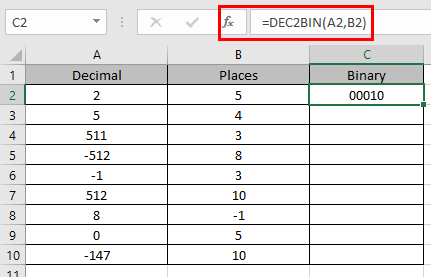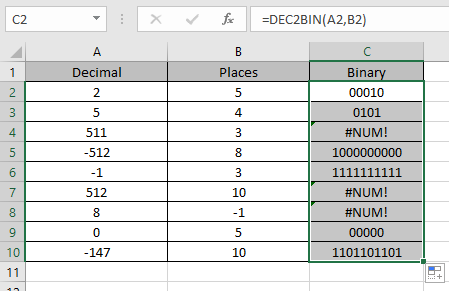# How to use the DEC2BIN Function in Excel

In this article, we will learn about how to use the DEC2BIN function in Excel.
DEC2BIN function in excel is used to convert decimal representation of numbers of radix 10 to binary  numbers (radix = 2).

The table shown below shows you some of the most used base & their radix of Alpha - numeric characters

 Base radix Alpha-Numeric Characters Binary 2 0 - 1 Octal 8 0 - 7 Decimal 10 0 - 9 hexadecimal 16 0 - 9 & A - F hexatridecimal 36 0 - 9 & A - Z

Binary number is representation of a number of radix 2. Only 2 digits are used in representation of a binary number 0 & 1. Where as decimal number representation have 10 digits from 0, 1, 2, 3, 4, 5, 6, 7, 8, 9 The below table will help you understand better

 Decimal Binary 0 000 1 001 2 010 3 011 4 100 5 101 6 110 7 111 8 1000

Successive division formulation is:

1. Successive division by 2 with the quotients.
2. Recording the remainder of the division from the last

Let’s take an example having a decimal value:
29 (decimal)

 Division quotient remainder 29 / 2 14 1 (last digit) 14 / 2 7 0 7 / 2 3 1 3 / 2 1 1 1 / 2 1 1 (first digit)

Reading from the last remainder values binary representation of 29 comes out to be : 11101 (upto 5 places)
The DEC2BIN function converts the decimal number of radix 10 to the binary number of radix 2.
Syntax:

=DEC2BIN ( number, [Places] )

Number : decimal number between - 512 to + 511 only integers.
[Places] : [optional] number where result expressed upto the number.

The input value to the function should be between -512 to 511. The resulting binary number will have upto 10 characters (10 bits), where first number express the sign of the number (positive or negative) & Other 9 express the value after the sign. ( 1 for negative & 0 for positive)To get the binary number, [places] number must have have sufficient places to express the binary number or else it returns the #NUM! Error. The decimal negative number is processed using two's complement notation.

Now let’s get more understanding of the function via using them in some examples.Here we have some binary values in Binary Column. We need to convert these binary numbers to decimal value.

Use the formula in Decimal column:

=DEC2BIN (A2, B2)

A2 : number provided to the function as cell reference
B2 : number provided to the function as cell reference

Values to the DEC2BIN function is provided as cell reference.The binary representation of 2 of base 10 (decimal) is 00010 of base 2 (decimal) upto 5 places.

Now copy the formula to other cells using the Ctrl + D shortcut key.As you can see here the DEC2BIN function returns the result of the input values.
Notes:

1. Numbers can be given as argument to the function directly without quotes or cell reference in excel.
2. The function doesn’t consider the [places] number in case of a negative decimal number.
3. The number must be a valid decimal number between - 512 to + 511.
4. If the input [places] number is not an integer, it is truncated by the function.
5. The function returns the binary value for the max value of + 511 & minimum value upto - 512 (only integers).
6. The function returns the #NUM! Error
1. If the input decimal number is less than - 512 or greater than + 511.
1. If the input number [places] is zero or negative.
2. If the input number [places] is not sufficient for the resulting positive binary number.
1. The function returns the #VALUE! Error
1. If the input number is text or non-numeric.
2. If the input [places] number is text or non-numeric.

Hope you understood how to use DEC2BIN function and referring cell in Excel. Explore more articles on Excel mathematical conversion functions here. Please feel free to state your query or feedback for the above article.

Related Articles:

How to use the DEC2BIN Function in Excel

How to use the BIN2OCT Function in Excel

How to use the BIN2HEX Function in Excel

How to use the DEC2OCT Function in Excel

How to use the DEC2HEX Function in Excel

How to use the OCT2BIN Function in Excel

How to use the OCT2DEC Function in Excel

How to use the OCT2HEX Function in Excel

How to use the HEX2BIN Function in Excel

How to use the HEX2OCT Function in Excel

How to use the HEX2DEC Function in Excel

Popular Articles:

If with wildcards

Vlookup by date

Join first and last name in excel

Count cells which match either A or B

Terms and Conditions of use

The applications/code on this site are distributed as is and without warranties or liability. In no event shall the owner of the copyrights, or the authors of the applications/code be liable for any loss of profit, any problems or any damage resulting from the use or evaluation of the applications/code.